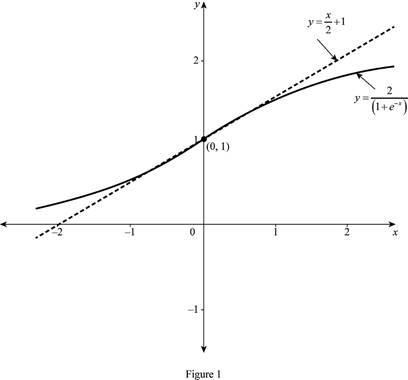# The equation of the tangent line to the curve at the point.### Single Variable Calculus: Concepts...

4th Edition
James Stewart
Publisher: Cengage Learning
ISBN: 9781337687805### Single Variable Calculus: Concepts...

4th Edition
James Stewart
Publisher: Cengage Learning
ISBN: 9781337687805

#### Solutions

Chapter 3.4, Problem 45E

(a)

To determine

## To find: The equation of the tangent line to the curve at the point.

Expert Solution

The equation of the tangent line to the curve y=2(1+ex) at (0,1) is y=x2+1.

### Explanation of Solution

Given:

The function is y=2(1+ex).

Derivative Rule: Quotient Rule

If f(x). and g(x) are both differentiable function, then

ddx[f(x)g(x)]=g(x)ddx[f(x)]f(x)ddx[g(x)][g(x)]2 (1)

Formula used:

The equation of the tangent line at (x1,y1) is, yy1=m(xx1) (2)

where, m is the slope of the tangent line at (x1,y1) and m=dydx|x=x1.

Calculation:

The derivative of y is dydx, which is obtained as follows,

dydx=ddx(y)=ddx(2(1+ex))

Apply the quotient rule as shown in equation (1),

ddx[2(1+ex)]=(1+ex)ddx2ddx[(1+ex)](1+ex)2=(1+ex)2(ddx(1)+ddx(ex))(1+ex)2=02(0+(ex))(1+ex)2=2(ex)(1+ex)2

Therefore, the derivative of y=2(1+ex) is dydx=2(ex)(1+ex)2_.

The slope of the tangent line at (0,1) is computed as follows,

m=dydx|x=02(e0)(1+e0)2    =2(1)(2)2       [Qe0=1]=12

Thus, the slope of the tangent line at (0,1) is m=12.

Substitute (0,1) for (x1,y1) and m=12 in equation (1),

(y1)=12(x0)y1=x2y=x2+1

Therefore, the equation of the tangent line to the curve y=2(1+ex) at (0,1) is y=x2+1.

(b)

To determine

Expert Solution

### Explanation of Solution

Given:

The equation of the curve is y=2(1+ex).

The equation of the tangent line is y=x2+1.

Graph:

Use the online graphing calculator to draw the graph of the functions as shown below in Figure 1.From the Figure 1, it is observed that the equation of the tangent line touches on the curve y=2(1+ex) at the point (0,1).

### Have a homework question?

Subscribe to bartleby learn! Ask subject matter experts 30 homework questions each month. Plus, you’ll have access to millions of step-by-step textbook answers!